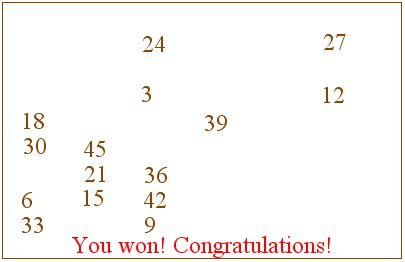# Euclid's Game

At the beginning of this simple game, the applet below displays a board with two numbers. At any time you can use the edit control to input a positive difference of any two numbers already present on the board. To do that, type in a number and press Enter. The loser is the player unable to make a move. If you wish the computer to move first, check the box "Please start".

As of 2018, Java plugins are not supported by any browsers (find out more). This Wolfram Demonstration, The Euclidean Algorithm, shows an item of the same or similar topic, but is different from the original Java applet, named 'euclid'. The originally given instructions may no longer correspond precisely.

The game is really very simple. It helps clarify the Euclid's algorithm and the notion of the Greatest Common Divisor of two integers. The difference of any two numbers is divisible by their $\gcd.\;$ Assuming the two original numbers are $N\;$ and $M\;$ and $N \gt M\;$ (In the applet they are never equal.) Then the only numbers that could be obtained by taking differences are the multiples of $\gcd (N, M).\;$ Furthermore, all such numbers will eventually appear on the board regardless of the sequence of moves (why?). Therefore, the total number of integers that will be written on the board equals $N/\gcd (N, M).\;$ From here you may calculate whether it's preferable to start or let the computer make the first move.

(The game may be played on a square grid, see a Java implementation for example.)

Assume the game is over - it's impossible to add a new difference. Let $a\;$ be the smallest number present. Then the collection of the numbers on the board coincides with the set $A\;$ of all multiples of $a\;$ not exceeding the largest of $M\;$ and $N.\;$ The proof is very similar to the way we established a fundamental property of $\gcd.\;$

First of all, both $a|N\;$ and $a|M.\;$ If, for example, $N = na + b,\;$ then we could form differences $N - a,\;$ $N - 2a,\;$ etc. and eventually get on the board $b \lt a\;$ which would contradict the minimality of $a.\;$ Therefore, for some $n\;$ and $m,\;$ $N=na\;$ and $M = ma.\;$ So what we have on the board is $A = \{ia:\;i = 1,\ldots, \max (n, m)\}.\;$ Now recollect that $a|\gcd (N, M)\;$. On the other hand, as we already remarked, the difference of any two numbers is divisible by their $\gcd.\;$ Therefore, any number on the board is divisible by $\gcd (N, M).\;$ In particular, $\gcd (N, M)|a.\;$ Therefore, $a = \gcd (N, M).\;$Another way to explain this stems directly from the Euclid's algorithm. Assuming $N \gt M,\;$ the first step is to form $N = sM + r.\;$ Note that, sooner or later, $r\;$ would appear on the board since it could be obtained by repeatedly subtracting $M\;$ first from $N\;$ and then from thus obtained numbers. The second step in the algorithm is to continue with $M = tr + u.\;$ Having both $M\;$ and $r\;$ on the board and proceeding with taking differences we will eventually get $u\;$ as well. Clearly we can now continue in this manner until the algorithms stops and $\gcd (N,M)\;$ has been found.